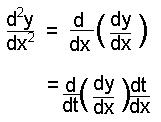## Parametric Differentiation

To differentiate parametric equations, we must use the chain rule.

Example

If x = 2at2 and y = 4at, find dy/dx

 dy = dy × dt dx dt dx

In this case, dx/dt = 4at and so dt/dx = 1/(4at)

Also dy/dt = 4a. Hence:
dy/dx = 4a × 1/4at = 1/t

Finding the Second Derivative

Finding the second derivative is a little trickier.

We use the fact that:Example

To find the second derivative in the above example, therefore:

d2y = d(1/t) × dt
dx2    dt         dx

= -1 ×  1 .
t2   4at